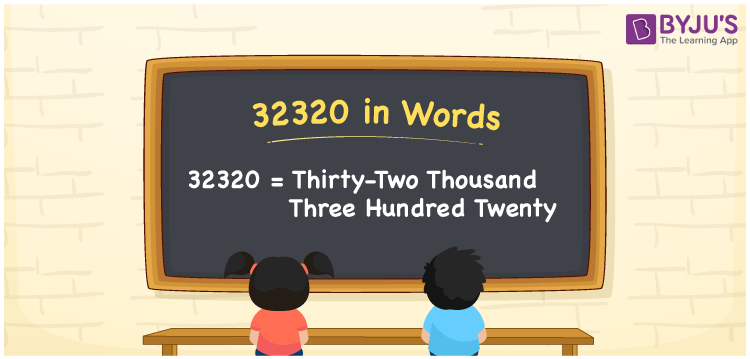# 32320 in Words

32320 in words is written as Thirty-two thousand three hundred twenty. In both the International System of Numerals and the Indian System of Numerals, 32320 is written as Thirty-two thousand three hundred twenty. The number 32320 is a Cardinal Number as it represents some quantity. For example, “that laptop costs 32320 rupees”.

 32320 in Words Thirty-two thousand three hundred twenty Thirty-two thousand three hundred twenty in Number 32320

## 32320 in English Words

32320 in English words is read as “Thirty-two thousand three hundred twenty.”## How to Write 32320 in Words?

To write 32320 in words, we shall use the place value chart. In the place value chart, put 3 in the ten thousands, 2 in the thousands, 3 in the hundreds, 2 in the tens and 0 in the ones. Let us make a place value chart to write the number 32320 in words.

 Ten Thousands Thousands Hundreds Tens Ones 3 2 3 2 0

Thus, we can write the expanded form as

3 × Ten Thousand + 2 × Thousand + 3 × Hundred + 2 × Ten + 0 × One

= 3 × 10000 + 2 × 1000 + 3 × 100 + 2 × 10 + 0 × 1

= 30000 + 2000 + 300 + 20 + 0

= 32320

= Thirty-two thousand three hundred twenty.

32320 is a natural number that is the successor of 32319 and the predecessor of 32321.

32320 in words – Thirty-two thousand three hundred twenty

• Is 32320 an odd number? – No
• Is 32320 an even number? – Yes
• Is 32320 a perfect square number? – No
• Is 32320 a perfect cube number? – No
• Is 32320 a prime number? – No
• Is 32320 a composite number? – Yes

## Frequently Asked Questions on 32320 in Words

### How to write 32320 in words?

32320 in words is written as Thirty-two thousand three hundred twenty.

### How to write 32320 in the International and Indian System of Numerals?

In both, the system of numerals, 32320 in words, is written as Thirty-two thousand three hundred twenty.

### How to write 32320 in a place value chart?

In the place value chart, write 3 in the ten thousands, 2 in the thousands, 3 in the hundreds, 2 in the tens and 0 in the ones.